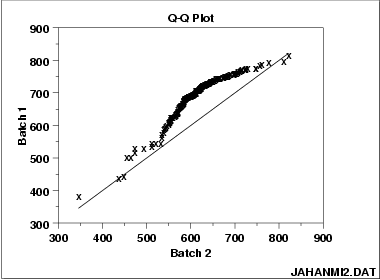jump to navigation

## The Quantile-Quantile Plot June 6, 2008

Posted by gordonwatts in statistics.
trackbackHas anyone used a “q-q” plot? How do they like it?

We often need to compare two histograms for similarity – we are curious to know if the two histograms are from the same source. Perhaps the most common time this occurs for us is comparing our Monte Carlo based background model to actual data. If we didn’t get the background model right we can’t go on and look for any signal!

We often have 100’s if not in the low 1000’s of these plots to compare. And we can’t really do it by eye – that introduces a human bias. So usually we resort to various statistical methods to compare them. The most common that I’m aware of is the so-called “K-S” test (Kolmogorov-Smirnov test). This produces a single number, and the nice thing is you can sort on that number and look at the worst cases and use those to guide you finding something incorrect.

Recently, on an internal discussion list, someone proposed the so-called “q-q” plot, sort for quantile-quantile plot. The plot attached to this blog-posting is an example. There are two batches of data, #1 along the vertical axis, and #2 along the horizontal access. Lets say that in sample 2, that 20% of the data is below 475, but in sample #1 20% of the data is below 550. Now do that for every % fraction (“quantile”). You can do this at all “%”‘s to make a plot as above. If the two samples were similar you might expect the point to fall along the 45 degree line (or on both sides of it due to statistical fluctuations).

I like this a lot better than a single number – you can tell what went wrong. The only disadvantage is you can’t sort by these plots and then look start by looking for the worst agreement. I wonder if you did something like the sum of the deviation from the 45 degree line if that would order in a way similar to the KS test?

Advertisements

## Comments»1. carlbrannen - June 7, 2008

Checking for deviation from 45 degrees reminds me of “non parametric statistics”, which was one of my favorite subject at my first grad school in math. The basic idea is to create statistical measures that do not depend on any assumed probability density for the underlying variables.

I don’t quite see how to apply this to the problem of comparing these sorts of objects, but I think it is worth reading about and letting it stew in the back of the head for a day or two. I’ll save a link to this post so If I think of something in the next day or two, I’ll add a comment.2. Zhiyi - June 12, 2008

Due to my understanding on KS test, it is an one-number version of QQ plot. Simply speaking, KS factor returns users a max distance of the empirical distribution function (ECDF) between 2 data sets, which, essentially, is a max deviation of qq plot from y=x. So I think we could use KS test factor to sort and use QQ plot to diagnose what is wrong with our background model. Since we always are working with histograms, both KS test and QQ plots cannot give us exact KS value and QQ plots defined standardly.

Since we are facing histograms, I think one thing needing to study is how to take error bars of distributions of 2 datasets into account of QQ plotting. This can tell us within uncertainty of datasets, how reliable the QQ plot is. For standard KS and QQ plot definitions, no such problem since their objects are value array instead of histograms.